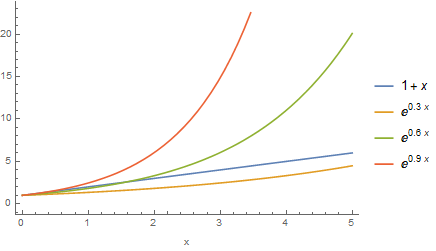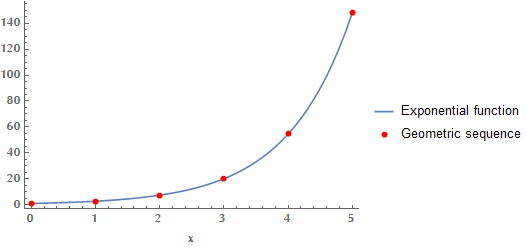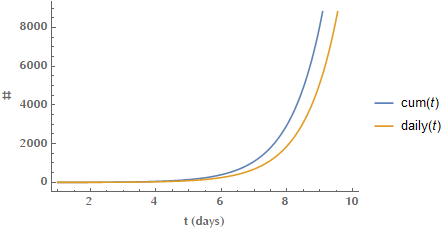Coronavirus disease 2019 (COVID-19) is an infectious disease caused by Severe Acute Respiratory Syndrome CoronaVirus 2 (SARS-CoV-2). At the time of writing Covid-19 has become a global pandemic. In many countries where lockdown was not enforced early on, coronavirus cases showed an exponential growth. After having talked to many people the last days, I came to understand that there are some common misconceptions regarding how an exponential function behaves. Here are my thoughts on this matter.

#### Misconception 1: Lack of fast growth, rules out exponential dynamics.

Many people think that exponential increase is a synonym for explosive, fast, immense growth. This may be true eventually, but for “small” values of $$x$$, an exponential function can be, initially, approximated by a linear function. This means that an exponential growth may masquerade as a linear function during the early phase of the phenomenon.

Why is that? Recall how $$\exp(x)$$ can be expressed as a Maclaurin series:

$\exp(x) = 1 + x + \frac{1}{2}x^2 + \frac{1}{6}x^3 + \ldots$

For “small” values of $$x$$, the contributions of higher order terms (those containing $$x^2$$, $$x^3$$, …) are minuscule. Therefore, in this case, the approximation $$\exp(x) \simeq 1 + x$$ holds. Similarly, if we take a look at $$\exp(\lambda x)$$, where the constant $$\lambda$$ tunes the growth rate, its Maclaurin series is:

$\exp(\lambda x) = 1 + \lambda x + \underbrace{\frac{1}{2}\lambda^2 x^2 + \frac{1}{6}\lambda^3 x^3 + \ldots}_{\text{Negligible for } \lambda\ll 1\text{ and small } x }$

Depending on the value of $$\lambda$$, the exponential function may trick us, for an extended period of time, into thinking that it’s linear. In the following image you may see how for different values of $$\lambda < 1$$, the respective functions hide their exponential nature for longer time (for larger values of $$x$$).Another example of an exponential change, that may span a very large amount of time, is the radioactive decay of Uranium-238. U-238 is the most common isotope of uranium found in nature and has a half-life of ~4.5 billion years. This means that the quantity of U-238 you have, will be reduced to its half after ~4.5 billion years. The mathematical formula describing this physical process is given by:

$N(t) = N_0 \exp(-\lambda t)$

Where $$t$$ is the elapsed time, $$N_0$$ is the initial quantity of the radioactive material (the material at $$t=0$$), $$\lambda$$ is the decay parameter (affecting how fast or slow the process is) and $$N(t)$$ is the quantity after time $$t$$ has elapsed. For U-238 the decay constant $$\lambda$$ is approximately equal to $$1.54 \times 10^{-10} \text{year}^{-1}$$.

#### Misconception 2: Exponential is “faster” than geometric

The difference between an exponential function and a geometric sequence is that the first takes continuous values, whereas the latter discrete. It doesn’t have anything to do with the rate of change.#### Misconception 3: The cumulative number of cases may be exponential, but the daily number of cases need not be exponential.

Let us assume that the cumulative number of cases up until time $$t$$ is given by the exponential formula: $$\text{cum}(t) = \exp(t)$$. Then, the number of daily cases at time $$t$$ can be calculated via the following logic:

\begin{align*} \text{cum}(t) = \exp(t) \Rightarrow \text{daily}(t) &= \text{cum}(t)-\text{cum}(t-1)\\ &= \exp(t) - \exp(t-1)\\ &= e^t \left(1 - \frac{1}{e}\right) = a e^t \end{align*}

So, the number of daily new cases is given by an exponential form as well. You can’t have exponentially increasing cumulative number of cases, without having exponential daily new cases.